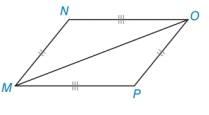Chapter 3.1, Problem 23E### Elementary Geometry for College St...

6th Edition
Daniel C. Alexander + 1 other
ISBN: 9781285195698

#### Solutions

Chapter
Section### Elementary Geometry for College St...

6th Edition
Daniel C. Alexander + 1 other
ISBN: 9781285195698
Textbook Problem
1 views

# In Exercises 21 to 24, the triangles named can be proved congruent. Considering the congruent pairs marked, name the additional pair of parts that must be congruent in order to use the method named.SSSΔ M N O ≅ Δ O P M

To determine

To prove:

The pair of parts in the triangles that must be congruent in order to use the SSS method to congruent the triangle.

Explanation

SSS is a method to find whether the triangle is congruent.

Here, S stands for side.

Given:

The given figure is,

Figure (1)

From figure (1),

MN¯OP¯

And,

MP¯ON¯

Approach:

Consider the triangles,

ΔMNO and ΔOPM.

Then, for the congruent of triangles the given method SSS must satisfy. As,

MN¯OP¯MP¯ON¯

For SSS method to satisfy all three sides of triangles must be congruent

### Still sussing out bartleby?

Check out a sample textbook solution.

See a sample solution

#### The Solution to Your Study Problems

Bartleby provides explanations to thousands of textbook problems written by our experts, many with advanced degrees!

Get Started